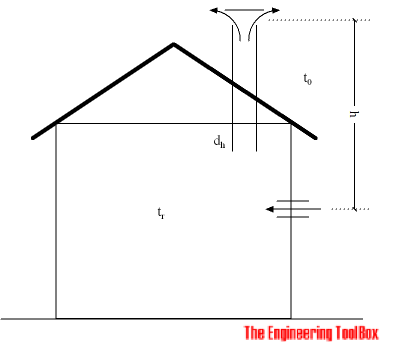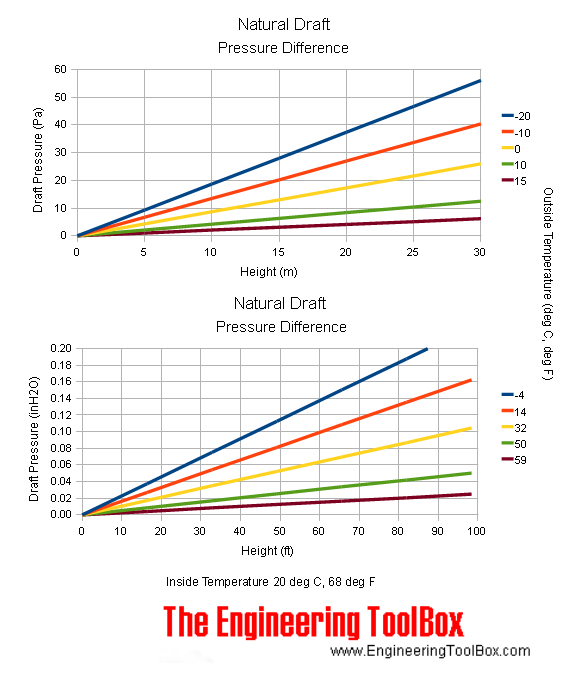Engineering ToolBox - Resources, Tools and Basic Information for Engineering and Design of Technical Applications!

# Natural Draft - Air Flow Volume and Velocity

## Air flow - volume and velocity - due to stack or flue effect caused by indoor hot and outdoor cold temperature difference.

The temperature difference between the outside air and the inside air creates a "natural draft" forcing air to flow through the building.

The direction of the air flow depends on the temperature of the outside and inside air. If the inside air temperature is higher than the outside air temperature, the inside air density is less than the outside air density, and the inside air will flow up and out of the upper parts of the building. The colder outside air will flow in to the lower parts of the building.

If the outside air temperature is higher than the inside air temperature - the inside air is more dense than the outside air - and the air flows down inside in the building. Warmer outside air flows in to the upper parts of the building.The natural draft head can be calculated as

dh mmH2O = 1000 h (ρ o - ρ r ) / ρ h2o (1)

where

dh mmH2O = head in millimeter water column (mm H 2 O)

ρ o = density outside air (kg/m 3 )

ρ r = density inside air (kg/m 3 )

ρ h2o = density water (in general 1000 kg/m 3 )

h = height between outlet and inlet air (m)

### Natural Draft Pressure

Equation (1) can be modified to SI pressure units:

dp = g ( ρ o - ρ r ) h                                   (1b)

where

d p = pressure (Pa, N/m 2 )

g = acceleration of gravity - 9.81 (m/s 2 )

### Density and Temperature

With air density 1.293 kg/m 3 at 0 o C - the air density at any temperature can be expressed as

ρ = (1.293 kg/m 3 ) (273 K) / (273 K + t)                                                 (2)

or

ρ = 353 / (273 + t) (2b)

where

ρ = density of air (kg/m 3 )

t = the actual temperature ( o C)

Equation (1) above can easily be modified by replacing the densities with equation (2) .

### Natural Draft Pressure Calculator

The calculator below can be used to calculate the natural draft pressure generated by the inside and outside temperature difference.

### Major and Minor System Loss

The natural draft force will be balanced to the major and minor loss in ducts , inlets and outlets. The major and minor loss in the system can be expressed as

dp = λ (l / d h ) ( ρ r v 2 / 2) + Σξ 1/2 ρ r v 2 (3)

where

dp = pressure loss (Pa, N/m 2 , lb f /ft 2 )

l = length of duct or pipe (m, ft)

d h = hydraulic diameter (m, ft)

Σ ξ = minor loss coefficient (summarized)

### Air Flow and Air Velocity

Equation (1) and (3) can be combined to express the air velocity through the duct

v = [(2 g ( ρ o - ρ r ) h ) / ( λ l ρ r / d h + Σ ξ ρ r )] 1/2 (4)

Equation (4) can also be modified to express the air flow volume through the duct

q = π d h 2 /4 [(2 g ( ρ o - ρ r ) h ) / ( λ l ρ r / d h + Σ ξ ρ r )] 1/2 (5)

where

q = air volume (m 3 /s)

### Natural Draft Air Flow and Velocity Calculator

The calculator below can be used to calculate the air flow volume and velocity in a duct similar to the drawing above. The friction coefficient used is 0.019 which is appropriate for normal galvanized steel ducts.

### Example - Natural Draft

Calculate the air flow caused by natural draft in a normal family house with two floors. The height of the hot air column from ground floor to outlet air duct above roof is approximately 8 m . The outside temperature is -10 o C and the inside temperature is 20 o C .

A duct of diameter 0.2 m goes from 1. floor to the outlet above the roof. The length of the duct is 3.5 m . Air leakages through the building are neglected. The minor coefficients are summarized to 1.

The density of the outside air can be calculated as

ρ o = (1.293 kg/m 3 ) (273 K) / ((273 K) + (-10 o C))

= 1.342 kg/m 3

The density of the inside air can be calculated as

ρ r = (1.293 kg/m 3 ) ( 273 K) / ((273 K) + (20 o C))

= 1.205 kg/m 3

The velocity through the duct can be calculated as

v = [(2 (9.81 m/s 2 ) ((1.342 kg/m 3 ) - (1.205 kg/m 3 )) (8 m)) / ( 0.019 (3.5m)(1.205 kg/m 3 )/(0.2 m) + 1 (1.205 kg/m 3 ) )] 1/2

= 3.7 m/s

The air flow can be calculated as

q = (3.7 m/s) 3.14 (0.2 m) 2 / 4

= 0.12 m 3 /s

### Note!

that these equations can be used for dry air, not for mass flow and energy loss calculations where air humidity may have vast effects.

### Natural Draft Chart - SI and Imperial Units## Related Topics

• ### Ventilation

Systems for ventilation and air handling - air change rates, ducts and pressure drops, charts and diagrams and more.

## Related Documents

• ### Air Curtains or Air Screens

Calculate air curtains or air screens in open doorways used to keep acceptable indoor comfort in buildings.
• ### Chimney Sizing

Calculate chimney draft and required chimney area - online calculator.
• ### Chimney's Size vs. Boiler Power

The size of chimney vs. boiler's heat load.
• ### Chimneys and Fireplace Sizing

Chimneys and fireplaces for stoves burning wood or coal as fuel.
• ### Colebrook Equation

Friction loss coefficients in pipes, tubes and ducts.
• ### Darcy-Weisbach Equation - Major Pressure and Head Loss due to Friction

The Darcy-Weisbach equation can be used to calculate the major pressure and head loss due to friction in ducts, pipes or tubes.
• ### Equivalent Length vs. Minor Pressure Head Loss in Pipe and Duct Components

Minor pressure and head loss in pipes vs. equivalent length in tubes and duct systems.
• ### HVAC Ducts - Air Velocities

Recommended air velocities in HVAC ducts.
• ### Infiltration - Heat Losses from Buildings

Estimated infiltration heat losses from buildings.
• ### Stack or Flue Effect

The stack or flue effect occurs when the outdoor temperature is lower than indoor temperature.

## Search

Search is the most efficient way to navigate the Engineering ToolBox.

## Engineering ToolBox - SketchUp Extension - Online 3D modeling!

Add standard and customized parametric components - like flange beams, lumbers, piping, stairs and more - to your Sketchup model with the Engineering ToolBox - SketchUp Extension - enabled for use with older versions of the amazing SketchUp Make and the newer "up to date" SketchUp Pro . Add the Engineering ToolBox extension to your SketchUp Make/Pro from the Extension Warehouse !

We don't collect information from our users. More about

## Citation

• The Engineering ToolBox (2003). Natural Draft - Air Flow Volume and Velocity. [online] Available at: https://www.engineeringtoolbox.com/natural-draught-ventilation-d_122.html [Accessed Day Month Year].

Modify the access date according your visit.

12.1.10